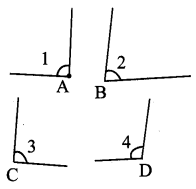# Samacheer Kalvi 9th Maths Solutions Chapter 4 Geometry Additional Questions

## Tamilnadu Samacheer Kalvi 9th Maths Solutions Chapter 4 Geometry Additional Questions

Exercise 4.1

Question 1.
Find the complement of each of the following angles
(i) 63°
(ii) 24°
(iii) 48°
Solution:
(i) The complement of 63° = 90° – 63° = 27°
(ii) The complement of 24° = 90° – 24° = 66°
(iii) The complement of 48° = 90° – 48° = 42°

Question 2.
Find the supplement of each of the following angles
(i) 58°
(ii) 148°
(iii) 120°
Solution:
(i) The supplement of 58° = 180° – 58° = 122°
(ii) The supplement of 148° = 180° – 148° = 32°
(iii) The supplement of 120° = 180° – 120° = 60°

Question 3.
Find the value of x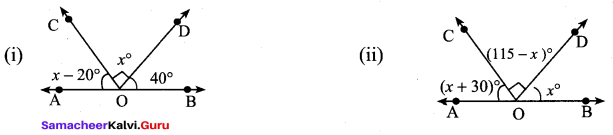Solution: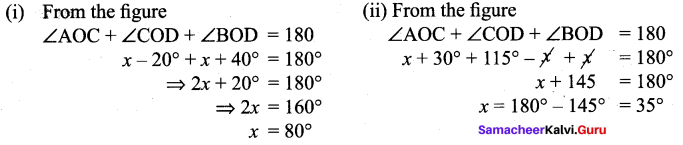Question 4.
Find the values of x, y in the following figures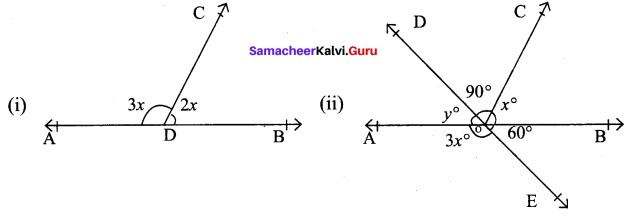Solution: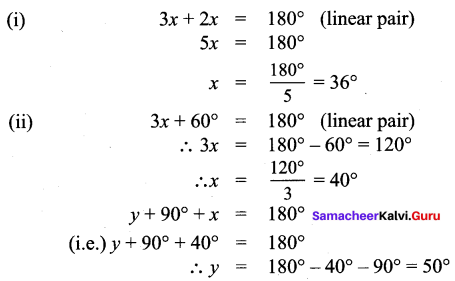Question 5.
In the given figure at right, side BC of ∆ABC is produced to D. Find ∠A and ∠C.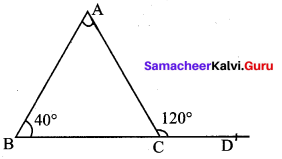Solution:
From the figure
Exterior angle = 120°
⇒ ∠C = 180° – 120° = 60° (linear pair)
∴ ∠A = 180° – (40° + 60°) = 80°

Exercise 4.2

Question 1.
If the measures of three angles of a quadrilateral are 100°, 84° and 76° then, find the measure of fourth angle.
Solution:
Let the measure of the fourth angle be x°.
The sum of the angles of a quadrilateral is 360°
So, 100° + 84° + 76° + x° = 360°
260° + x° = 360°
x = 360° – 260° = 100°
Hence, the measure of the fourth angle is 100°.

Question 2.
In the parallelogram ABCD if ∠A = 65°, find ∠B, ∠C and ∠D.
Solution:
Let ABCD be a parallelogram in which ∠A = 65°
Since AD || BC we can treat AB as a transversal. So
∠A+∠B = 180°65° +∠B = 180°
∠B = 180°-65°
∠B = 115°
Since the opposite angles of a parallelogram are equal, we have
∠C = ∠A = 65° and ∠D = ∠B = 115°
Hence, ∠B = 115°, ∠C = 65° and ∠D = 115°

Question 3.
If ABCD is a rhombus and if ∠A = 76°, find ∠CDB.
Solution:
∠A = ∠C = 76° (Opposite angles of a rhombus)
Let ∠CDB = x°. In ∆CDB, CD = CB
∠CDB + ∠CBD + ∠DCB = 180° (Angles of a triangle)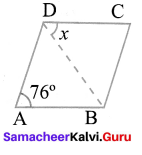2x° + 76° = 180° ⇒ 2x° = 104°
x° = 52°
∴ ∠CDB = 52°

Question 4.
In a parallelogram, opposite sides are equal
Solution:
Given ABCD is a parallelogram
To Prove ABCD and DA = BC
Construction Join AC
Proof
Since ABCD is a parallelogramAD || BC and AC is the transversal
∠DAC = ∠BCA ➝ (1) (alternate angles are equal)
AB || DC and AC is the transversal
∠BAC = ∠DCA ➝ (2) (alternate angles are equal)
∠DAC = ∠BCA from (1)
AC is common
∠DCA = ∠BAC from (2)
Hence AD = CB and DC = BA (Corresponding sides are equal)

Question 5.
The angles of a quadrilateral are ¡n the ratio 1 : 2 : 3 : 4. Find all the angles. Let each ratio be x.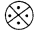Solution:
Then the angles are x°, 2x°, 3x°, 4x°
x° + 2x° + 3x° + 4x° = 360°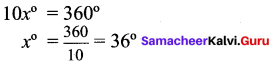Exercise 4.3

Question 1.
The radius of a circle 15 cm and the length of one of its chord is 24 cm. Find the distance of the chord from the centre.
Solution:
Distance of the chord from the centre.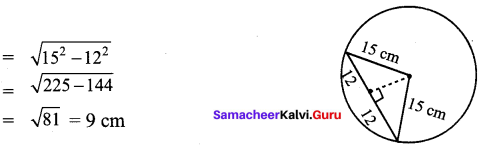Question 2.
The chord of length 32 cm is drawn at the distance of 12 cm from the centre of the circle. Find the radius of the circle.
Solution: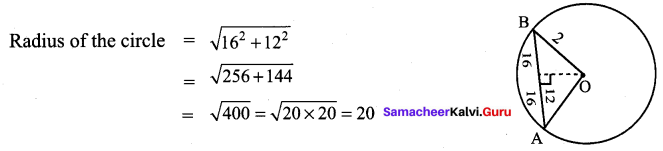Question 3.
In a circle, AB and CD are two parallel chords with centre O and radius 5 cm such that AB = 8 cm and CD = 6 cm determine the distance between the chords?
Solution: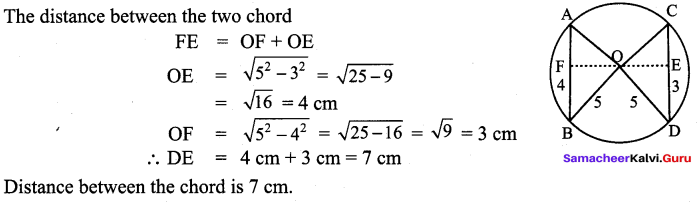Question 4.
Find the value of x°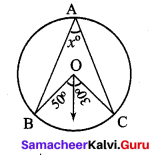Solution: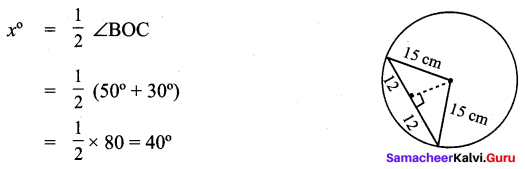Question 5.
Find the value of x°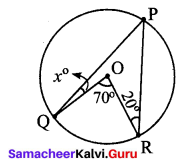Solution: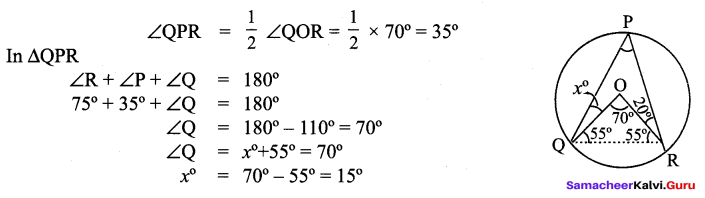Exercise 4.4

Question 1.
Find the value of x in the figure.
Solution: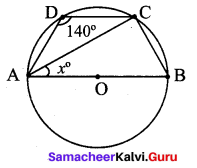∠ABC – 180°- 140° = 40°
∠BCA = 90°
∴ x = ∠BAC = 180°- (90° + 40°) = 50°

Question 2.
Find all the angles of the given cyclic quadrilateral ABCD in the figure.Solution: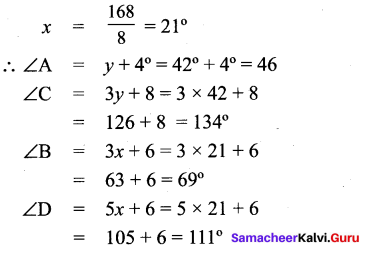Question 3.
AB and CD are two parallel sides of a cyclic quadrilateral ABCD in the figure. such that AB = 12 cm, CD = 16 cm and the radius of the circle is 10cm. Find the shortest distance between the two sides AB and CD.
Solution:
In this figure,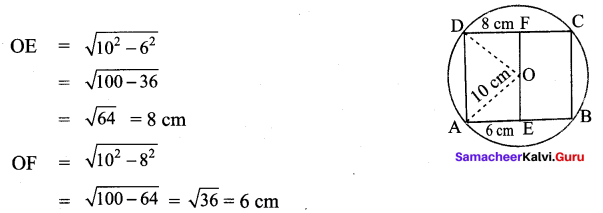The shortest distance between the two sides = 8 + 6 = 14 cm

Question 4.
In the given figure, AB and CD are the parallel chords of a circle with centre O, such that AB = 30 cm and CD = 40 cm. If OM ⊥ AB and OL ⊥ CD distance between LM is 35 cm. Find the radius of the circle?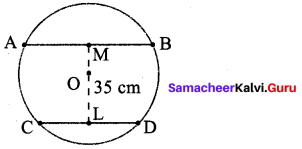Solution: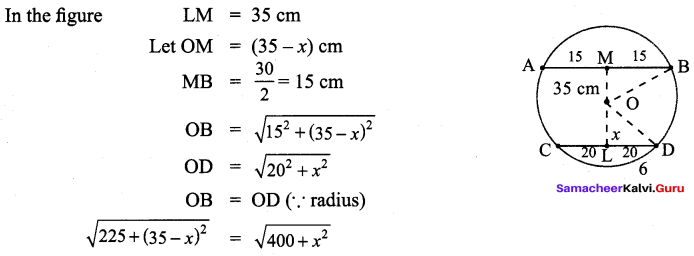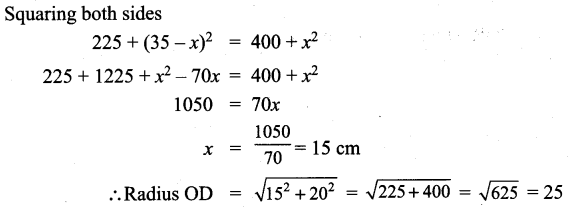Exercise 4.5

Question 1.
Construct an equilateral triangle of sides 6 cm and locate its orthocentre.
Solution: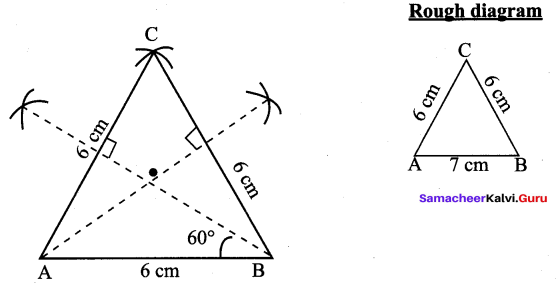Construction:
(1) Draw the ∆ABC with the given measurements.
(2) Construct the altitudes from any two vertices (A and B) to their opposite sides BC and AC respectively.
(3) The point of intersection of the altitudes H is the orthocentre of the given ∆ABC.

Question 2.
Draw and locate the orthocentre of a right triangle PQR right angled at Q, with PQ = 4.5 cm and QR = 6 cm.
Solution: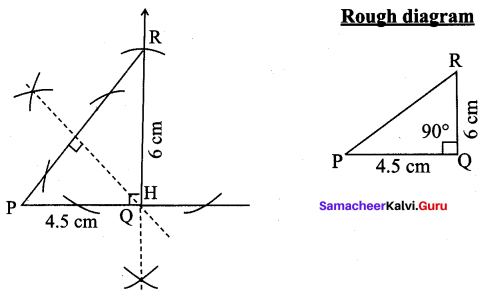Construction:
(1) Draw the ∆PQR with the given measurements.
(2) Construct altitudes from any two vertices (Q and R) to their opposite sides PR and PQ respectively.
(3) The point of intersection of the altitudes H is the orthocentre of the given ∆PQR.

Question 3.
Construct the circumcentre of the ∆ABC with AB = 5 cm, ∠A = 60° and ∠B = 80°, also draw two circumcircle and find the circum radius of the ∆ABC.
Solution: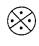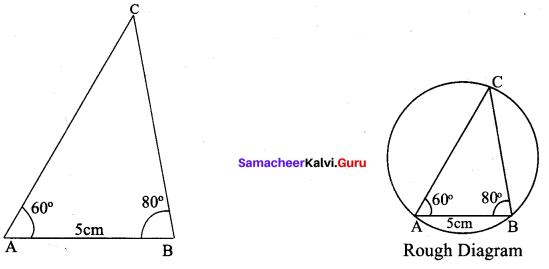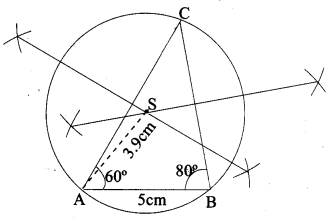Solution:
Step 1: Draw the ∆ABC with the given measurements.
Step 2 : Construct the perpendicular bisector of any two sides (AC and BC) and let them meet at S which is the circumcentre.
Step 3 : S as centre and SA = SB = SC as radius, draw the Circumcircle to passes through A,B and C. Circumradius = 3.9 cm.

Exercise 4.6

Question 1.
Draw the circumcircle for an equilateral triangle of side 6 cm.
Solution: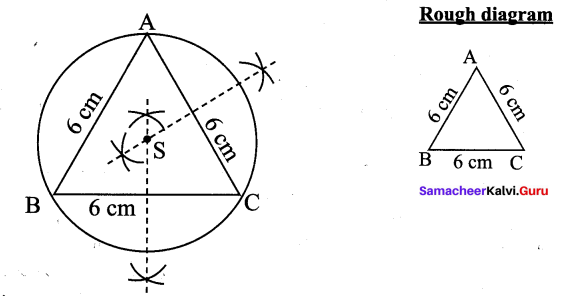Construction:
(1) Draw the ∆ABC with the given measurements.
(2) Construct the perpendicular bisectors of AC and BC and let them meet at S which is the circumcentre.
(3) With S as centre and SA = SB = SC as radius, draw the circumcircle to pass through A, B and C.

Question 2.
Construct the centroid of ∆PQR such that PQ = 9 cm, PQ = 7cm, RP = 8 cm.
Solution:
In ∆PQR,
PQ = 5 cm,
PR = 6 cm
∠QPR = 60°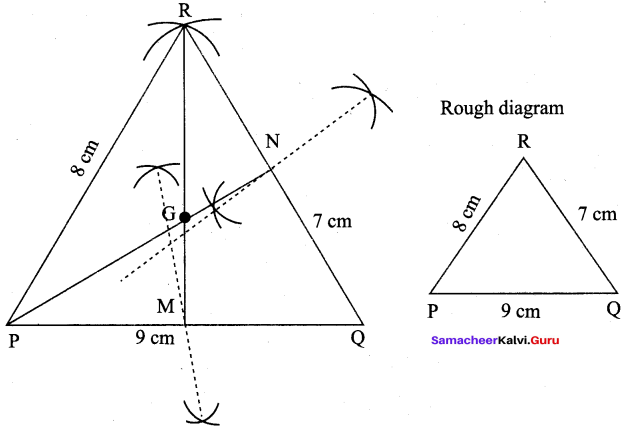Construction :
Step 1 : Draw ∆PQR using the given measurements PQ = 9 cm, QR = 7 cm and RP = 8 cm and construct the perpendicular bisector of any two sides (PQ and QR) to find the mid-points M and N of PQ and QR respectively.
Step 2 : Draw the medians PN and RM and let them meet at G. The point G is the centroid of the given ∆PQR.

Question 3.
Draw and locate the centroid of the triangle ABC where right angle at A, AB = 8 cm and AC = 6 cm.
Solution: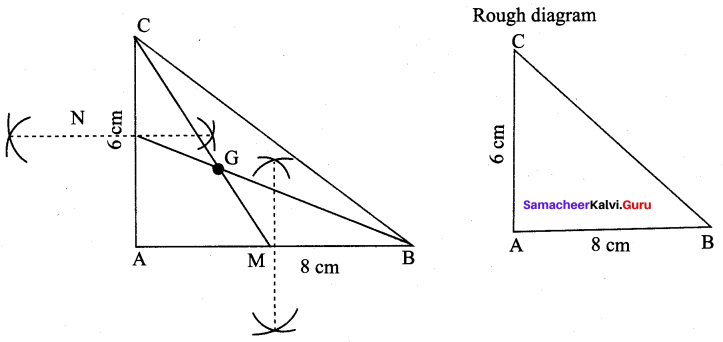Step 1 : Draw ∆ABC with the given measurements AB = 8 cm, ∠A = 90° and AC = 6 cm and construct the perpendicular bisector of any two sides (AB and AC) to find the mid points M and N of AB and BC respectively.
Step 2 : Draw the medians (C and BN and let them meet at G. The point G is the centroid of the given ∆ABC.

Question 4.
Construct the centroid of Ø PQR whose sides are PQ = 8 cm, QR = 6 cm, RP = 7 cm.Solution:
Side = 6.5 cm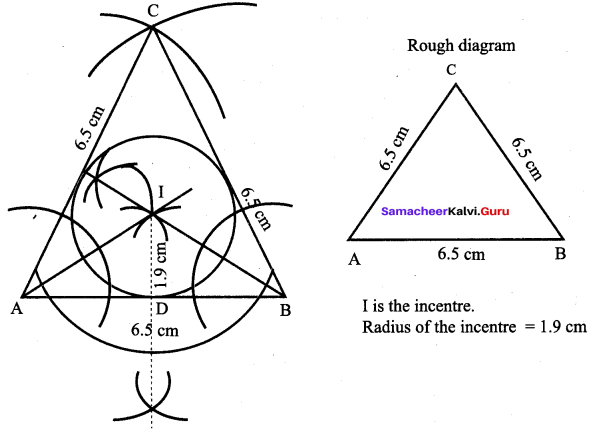Construction:
Step 1 : Draw ∆ABC with AB = BC = CA = 6.5 cm
Step 2 : Construct angle bisectors of any two angles (A and B) and let them meet at 1.1 is the incentre of ∆ABC.
Step 3 : Draw perpendicular from I to any one of the side (AB) to meet AB at D.
Step 4 : With I as centre, ID as radius draw the circle. This circle touches all the sides of triangle internally.

Exercise 4.7

Multiple Choice Questions :

Question 1.
If an angle is equal to one third of its supplement, its measure is equal to
(1) 40°
(2) 50°
(3) 45°
(4) 55°
Hint: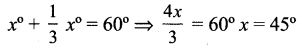Solution:
(3) 45°

Question 2.
In the given figure, OP bisect ∠BOC and OQ bisect ∠AOC. Then ∠POQ is equal to
(1) 90°
(2) 120°
(3) 60°
(4) 100°
Hint: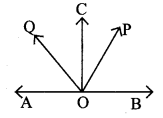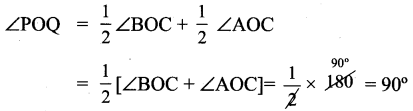Solution:
(1) 90°

Question 3.
The complement of an angle exceeds the angle by 60°. Then the angle is equal to
(1) 25°
(2) 30°
(3) 15°
(4) 35°
Hint: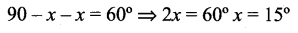Solution:
(3) 15°

Question 4.
ABCD is a parallelogram, E is the mid-point of AB and CE bisects ∠BCD. Then ∠DEC is
(1) 60°
(2) 90°
(3) 100°
(4) 120°
Solution:
(2) 90°

Question 5.
If the length of a chord decreases, then its distance from the centre.
(1) increases
(2) decreases
(3) same
(4) cannot say
Solution:
(1) increases

Question 6.
In the figure, O is the centre of the circle and ∠ACB = 60° then ∠AOB =
(1) 60°
(2) 90°
(3) 120°
(4) 180°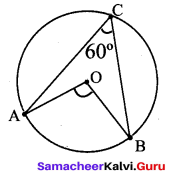Solution:
(3) 120°

Question 7.
The angle subtend by a semicircle at the centre is.
(1) 60°
(2) 90°
(3) 120°
(4) 180°
Solution:
(4) 180°

Question 8.
The angle subtend by a semicircle at the remaining part of the circumference is ___.
(1) 60°
(2) 90°
(3) 120°
(4) 180°

Text Book Activities

Activity – 3

Angle sum for a polygon.
Mark a point P in its interior. Join the segments PA, PB, PC and PD.
You have 4 triangles now.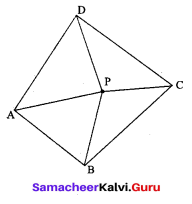How much is the sum of all the angles of the 4 triangles?
How much is the sum of the angles at their vertex, now P?
Can you now find the ‘angle sum’ of the quadrilateral ABCD?
Can you extend this idea to any polygon?
Solution:
Sum of the angles of the 4 triangle = 180° × 4 = 720°
Sum of the angles at their vertex, now p = 360°
Angle sum of the quadrilateral ABCD = 720°- 360° = 360°
Yes we can extend this idea to any polygon.

Activity – 4

Procedure.

1. Draw a circle with centre O and with suitable radius.
2. Make it a semi-circle through folding. Consider the point A, B on it.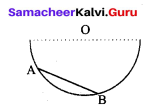3. Make crease along AB in the semi circles and open it.
4. We get one more crease line on the another part of semi circle, name it as CD (observe AB = CD)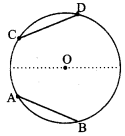5. Join the radius to get the ∆OAB and ∆OCD.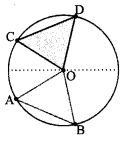6. Using trace paper, take the replicas of triangle ∆OAB and ∆OCD.
7. Place these triangles ∆OAB and ∆OCD one on the other.

Activity – 6
Procedure:
1. Draw a circle of any radius with centre O.
2. Mark any four points A, B, C and D on the boundary. Make a cyclic quadrilateral ABCD and name the angles as in figure.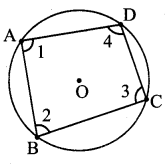3. Figure Make a replica of the cyclic quadrilateral ABCD with the help of tracing paper.
4. Make the cutout of the angles A, B, C and D
5. Paste the angle cutout ∠1, ∠2, ∠3 and ∠4 adjacent to the angles opposite to A, B, C and D as in Figure.
6. Measure the angles ∠1 + ∠3, and ∠2 + ∠4.
Solution:
∠1 + ∠3 = 180°
∠2 + ∠4 = 180°